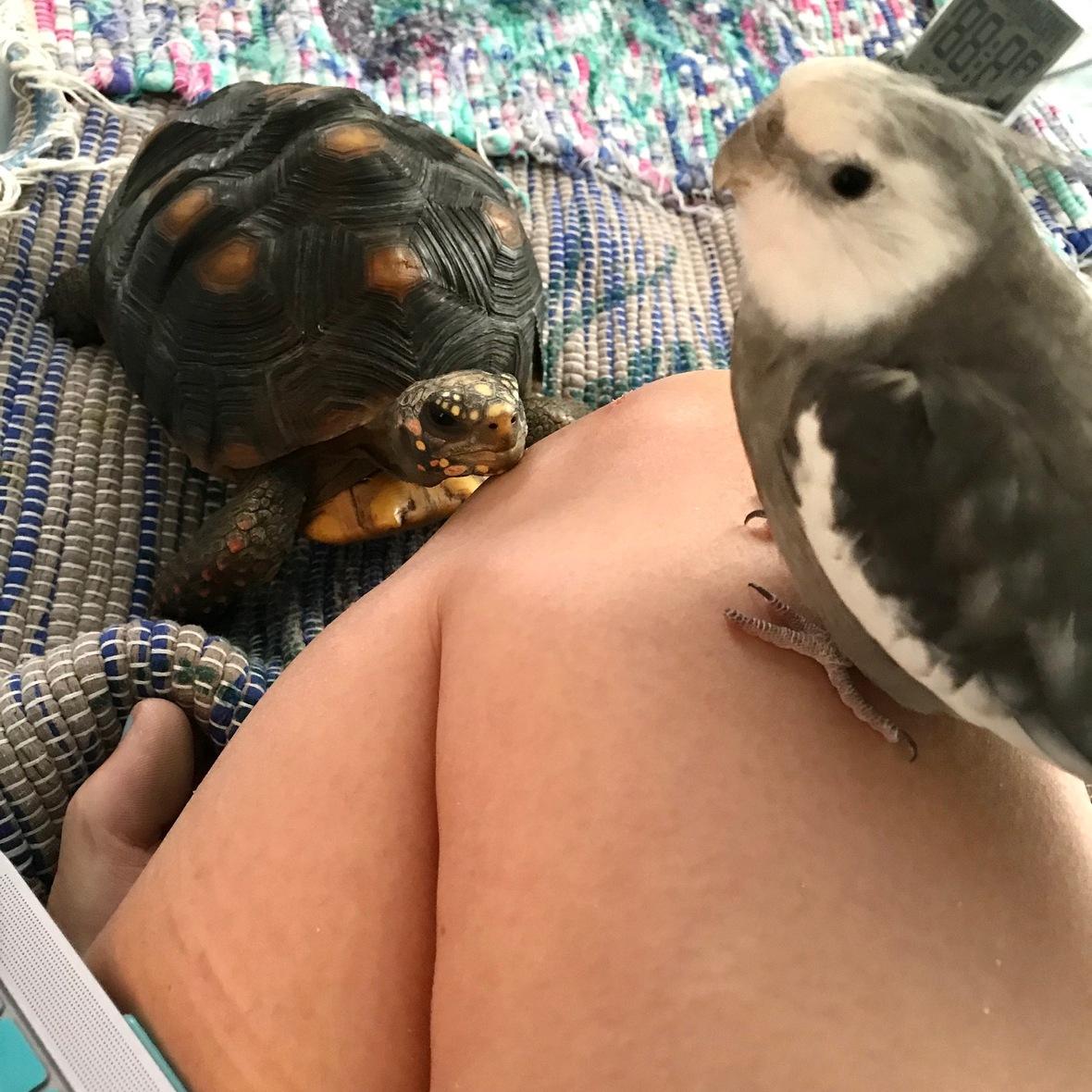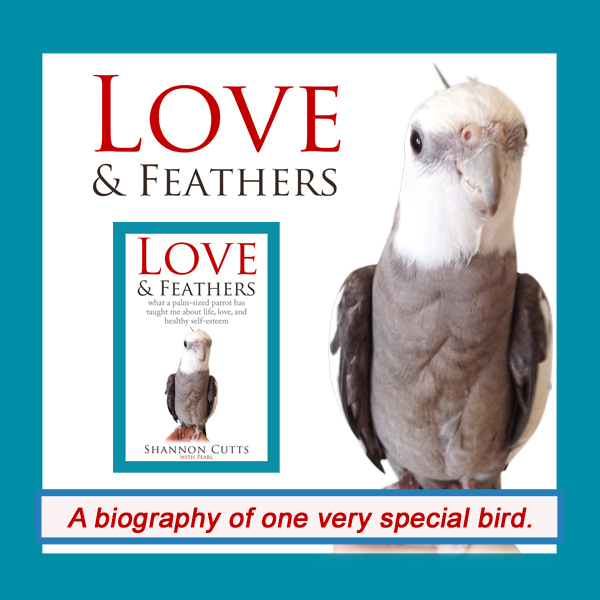# August 2018

 table div table+table+table div table{width:100%;padding:0}table div table+table+table div table img{width:96.23%;padding:0;float:none}table div table+table+table div table td{width:100%;padding:0 1.88% 18px}/* styles *//* styles */ Receiving this email as a forward? Sign up to keep following our little flock's adventures!
 table div table+table+table+table+table+table div table{width:100%;padding:0}table div table+table+table+table+table+table div table img{width:96.23%;padding:0;float:none}table div table+table+table+table+table+table div table td{width:100%;padding:0 1.88% 18px}/* styles */## Waiting = BraveEven as I was typing out my thoughts here, Malti (my young tortoise) and Pearl (my parrot) were having the most interesting "what if" simulation, with Malti gazing at Pearl and Pearl gazing at me and my brain/mind thinking it had no idea how to simulate in advance how this interaction might go....

Saving money. Healing the body. Healing the mind. Falling in love. Grieving a loss. Getting in shape. Forgiving a hurt. Choosing the half-full glass.

Sometimes the time it takes for the next steps in life to unfold is just a lightning-bug flash - the tiniest instant of instants.

And sometimes, the time it takes to get from where we are now to where we are going next is a lifetime....or it feels like it, anyway.

Of course, just permitting each new experience, each unfolding process, to begin at all also requires patience (lots of it) and tremendous courage.

Which is why waiting = brave in my book.

Happily, it seems I'm not alone in this interpretation. In a chapter compellingly called "Why a Brain?," What It's Like to Be a Dog author Gregory Berns talks about why dogs (and people) have brains.

He says it is so we can learn.

Dr. Berns talks about how in nature - like in the food chain part of nature which we homo sapiens used to participate in daily but only occasionally visit these days - there are no do-overs. And when you can't opt out of inhabiting your rank and position in the natural food chain, you need to find a way to "practice" not getting eaten....preferably well before such a situation actually arises.

From his studies of how brains work, Dr. Berns concludes that one of the best ways to practice not getting eaten in advance is to run simulations.

Specifically, Dr. Berns explains, our brains have evolved to run "what if" simulations - sort of like how we would strategize mentally if we were trying to win at a game of chess. We would study our opponent, the board itself, the pieces (ours and theirs), visualizing different maneuvers to ourselves until we find the one that appears most likely to deliver a victory.

Then, having simulated our way to a strategy that seems most personally advantageous, we would finally make our move. And then we would hope like crazy that we win and don't die so we can learn some more tomorrow.

But there is a bit of wrinkle even in this time-tested process of staying alive.

Because my body may be waiting. My heart may be waiting. My soul may even be waiting (and since it is my soul and not the rest of me, it might even be waiting somewhat patiently).

But my brain - my mind - is not waiting. Oh no. As previously mentioned in detail, my brain/mind is oh-so-busy running "what if" simulations.

It is working so furiously and so fast to come up with all these simulations, all of these "what if" scenarios, to attempt to help me win and not die in the future while I wait (bravely, I hope) in the present.

Not surprisingly, since the point of all these simulations is to avoid death, many of the proposed scenarios are somewhat....grim.

Like, I might wind up penniless and alone living on the streets. Now what?

Or, I might get left, again, by the love of my life, and have my heart broken, again, into a million-zillion pieces. Now what?

Or, my health might deteriorate to the point where I am bedridden or in a coma and then my medical insurance might run out. Now what?

So waiting takes tremendous bravery, at least where my personal brain's balls-to-the-wall all-too-realistic life-or-death simulations are concerned.

I do understand my brain/mind is showing me love in its own odd and terrifying way. It wants me to stay alive. It wants to stay alive. It wants us to stay alive and be together in the future.

But in the present, it is seriously bumming me out, not to mention scaring me to, well, death.

(Here, it makes sense how cortisol (aka "the stress hormone") researchers say too much stress can literally kill you.)

So what does it mean - and what is my point - that it takes the time it takes?

My basic point is that worrying about the time whatever-it-is is taking never seems to change the time it actually takes, at least in my little world. Worrying just doesn't change anything.

And I can't keep my brain from running its nonstop fight-or-flight survivalist simulations while the time it takes creeps on and on and on.

What I can do is take a step back - several steps back if I'm paying attention and the simulation is particularly gripping - and say two things to myself:

1. It takes the time it takes. Hurrying or stalling or attempting to opt out won't change this.
2. That (scary thing my mind is thinking right now) is just a brain simulation - a "what if" scenario - it is not yet "real" and thus cannot hurt or help me.
 1 It takes the time it takes. Hurrying or stalling or attempting to opt out won't change this.
 2 That (scary thing my mind is thinking right now) is just a brain simulation - a "what if" scenario - it is not yet "real" and thus cannot hurt or help me.

By reminding myself of these two things, I get a bit of distance from my own very-much-present fear and impatience.

Specifically, I remind my soul that life in a physical body absolutely does feel this ponderous and plodding and that this is normal and unavoidable as long as the body is along for the ride.

I remind my heart that "things taking time" doesn't mean "never" - it takes the time it takes, but it WILL happen (whatever it is that needs to happen).

I remind my emotions that the fear, too, will pass. It will. And there are other - happier - emotions that may pop up at any moment, so just wait for it.

Together, "we" [body, brain/mind, emotions, heart, spirit] wait. We are not alone in this. We are together.

With great respect and love,

Shannon

 table div table+table+table+table+table+table+table+table+table div table{width:100%;padding:0}table div table+table+table+table+table+table+table+table+table div table img{width:96.23%;padding:0;float:none}table div table+table+table+table+table+table+table+table+table div table td{width:100%;padding:0 1.88% 18px}/* styles */# Get your copy of Love & Feathers the book!Click on the image of Pearl to order your signed copy!Pearl will even beak-o-graph it for you!
 table div table+table+table+table+table+table+table+table+table+table+table+table+table+table div table{width:100%;padding:0}table div table+table+table+table+table+table+table+table+table+table+table+table+table+table div table img{width:96.23%;padding:0;float:none}table div table+table+table+table+table+table+table+table+table+table+table+table+table+table div table td{width:100%;padding:0 1.88% 18px}/* styles */# Malti displaying her "basking" skills!

 table.module-15{width:73.02%;padding:0}table div table+table+table+table+table+table+table+table+table+table+table+table+table+table+table+table div table{width:73.02%;float:none;margin-left:auto;margin-right:auto;padding:0}table div table+table+table+table+table+table+table+table+table+table+table+table+table+table+table+table div table a{border:0 none;text-decoration:none}table div table+table+table+table+table+table+table+table+table+table+table+table+table+table+table+table div table img{width:100%!important;border:0 none;text-decoration:none}table div table+table+table+table+table+table+table+table+table+table+table+table+table+table+table+table div table td{width:100%;padding:0}/* styles */
 table div table+table+table+table+table+table+table+table+table+table+table+table+table+table+table+table+table+table div table{width:100%;padding:0}table div table+table+table+table+table+table+table+table+table+table+table+table+table+table+table+table+table+table div table img{width:96.23%;padding:0;float:none}table div table+table+table+table+table+table+table+table+table+table+table+table+table+table+table+table+table+table div table td{width:100%;padding:0 1.88% 18px}/* styles */# Summer clearly agrees with Bruce!

 table.module-19{width:73.02%;padding:0}table div table+table+table+table+table+table+table+table+table+table+table+table+table+table+table+table+table+table+table+table div table{width:73.02%;float:none;margin-left:auto;margin-right:auto;padding:0}table div table+table+table+table+table+table+table+table+table+table+table+table+table+table+table+table+table+table+table+table div table a{border:0 none;text-decoration:none}table div table+table+table+table+table+table+table+table+table+table+table+table+table+table+table+table+table+table+table+table div table img{width:100%!important;border:0 none;text-decoration:none}table div table+table+table+table+table+table+table+table+table+table+table+table+table+table+table+table+table+table+table+table div table td{width:100%;padding:0}/* styles */
 table div table+table+table+table+table+table+table+table+table+table+table+table+table+table+table+table+table+table+table+table+table+table div table{width:100%;padding:0}table div table+table+table+table+table+table+table+table+table+table+table+table+table+table+table+table+table+table+table+table+table+table div table img{width:96.23%;padding:0;float:none}table div table+table+table+table+table+table+table+table+table+table+table+table+table+table+table+table+table+table+table+table+table+table div table td{width:100%;padding:0 1.88% 18px}/* styles */## Meet Pearl, Malti, Bruce & Me

 table div table+table+table+table+table+table+table+table+table+table+table+table+table+table+table+table+table+table+table+table+table+table+table+table div table,table.module-23{width:34.34%;float:left;padding:0}table div table+table+table+table+table+table+table+table+table+table+table+table+table+table+table+table+table+table+table+table+table+table+table+table div table a{border:0 none;text-decoration:none}table div table+table+table+table+table+table+table+table+table+table+table+table+table+table+table+table+table+table+table+table+table+table+table+table div table img{width:100%!important;border:0 none;text-decoration:none}table div table+table+table+table+table+table+table+table+table+table+table+table+table+table+table+table+table+table+table+table+table+table+table+table div table td{width:100%;padding:0 20px 20px 0}/* styles */ Hi! It is so good to meet you here each month! I'm so excited about our new book, "Love & Feathers: what a palm-sized parrot has taught me about life, love, and healthy self-esteem."The book focuses on the power of animal mentors in our lives and features my parrot, Pearl. Malti is a young red-foot tortoise with a healthy appetite for food, snoozing and life! Bruce, a rescued Texas 3-toed box turtle, is our newest shelled flock member. My first book, "Beating Ana: how to outsmart your eating disorder and take your life back," is a practical hands-on workbook to help overcome eating disorders as well as the story of my personal recovery journey. MentorCONNECT, the first global eating disorders nonprofit mentoring community, emerged from the experience of writing "Beating Ana." While we closed in April 2017, the website continues to provide information about mentoring-based recovery support resources. ==> To connect with me: www.shannoncutts.com ==> To connect with Pearl, Malti & Bruce: www.loveandfeathersandshells.com
 table div table+table+table+table+table+table+table+table+table+table+table+table+table+table+table+table+table+table+table+table+table+table+table+table+table div table{width:100%;padding:0}table div table+table+table+table+table+table+table+table+table+table+table+table+table+table+table+table+table+table+table+table+table+table+table+table+table div table img{width:96.23%;padding:0;float:none}table div table+table+table+table+table+table+table+table+table+table+table+table+table+table+table+table+table+table+table+table+table+table+table+table+table div table td{width:100%;padding:0 1.88% 18px}/* styles */table div table+table+table+table+table+table+table+table+table+table+table+table+table+table+table+table+table+table+table+table+table+table+table+table+table+table div table td,table.module-25{width:100%;padding:0}table div table+table+table+table+table+table+table+table+table+table+table+table+table+table+table+table+table+table+table+table+table+table+table+table+table+table div table{width:100%;float:none;margin-left:auto;margin-right:auto;padding:0}table div table+table+table+table+table+table+table+table+table+table+table+table+table+table+table+table+table+table+table+table+table+table+table+table+table+table div table a{border:0 none;text-decoration:none}table div table+table+table+table+table+table+table+table+table+table+table+table+table+table+table+table+table+table+table+table+table+table+table+table+table+table div table img{width:100%!important;border:0 none;text-decoration:none}/* styles */
 table.module-26{width:74.15%;padding:0}table div table+table+table+table+table+table+table+table+table+table+table+table+table+table+table+table+table+table+table+table+table+table+table+table+table+table+table div table{width:74.15%;float:none;margin-left:auto;margin-right:auto;padding:0}table div table+table+table+table+table+table+table+table+table+table+table+table+table+table+table+table+table+table+table+table+table+table+table+table+table+table+table div table a{border:0 none;text-decoration:none}table div table+table+table+table+table+table+table+table+table+table+table+table+table+table+table+table+table+table+table+table+table+table+table+table+table+table+table div table img{width:100%!important;border:0 none;text-decoration:none}table div table+table+table+table+table+table+table+table+table+table+table+table+table+table+table+table+table+table+table+table+table+table+table+table+table+table+table div table td{width:100%;padding:0}/* styles */
 table div table+table+table+table+table+table+table+table+table+table+table+table+table+table+table+table+table+table+table+table+table+table+table+table+table+table+table+table div table{width:100%;padding:0}table div table+table+table+table+table+table+table+table+table+table+table+table+table+table+table+table+table+table+table+table+table+table+table+table+table+table+table+table div table img{width:96.23%;padding:0;float:none}table div table+table+table+table+table+table+table+table+table+table+table+table+table+table+table+table+table+table+table+table+table+table+table+table+table+table+table+table div table td{width:100%;padding:0 1.88% 18px}/* styles */## Reprints & Reposts

REPRINTS: Would you like to use any portion of this material in your newsletter, blog or website? You may do so as long as you also include this copyright information: